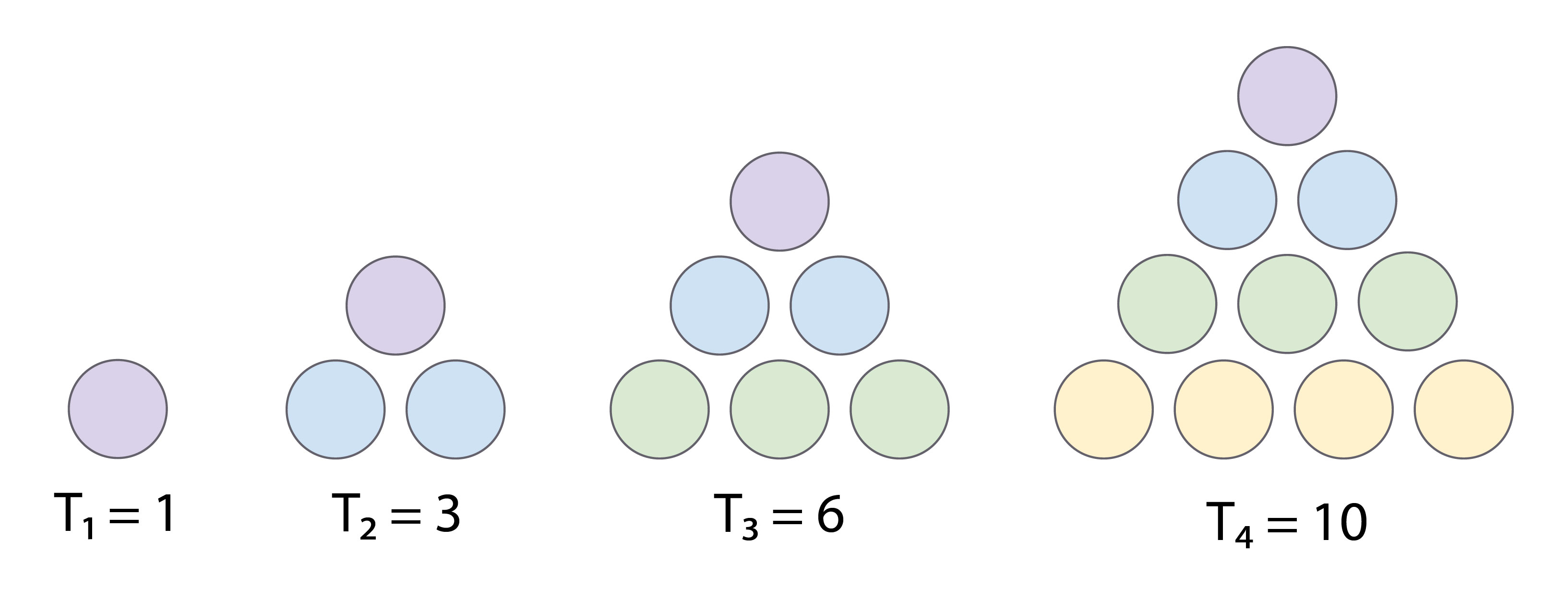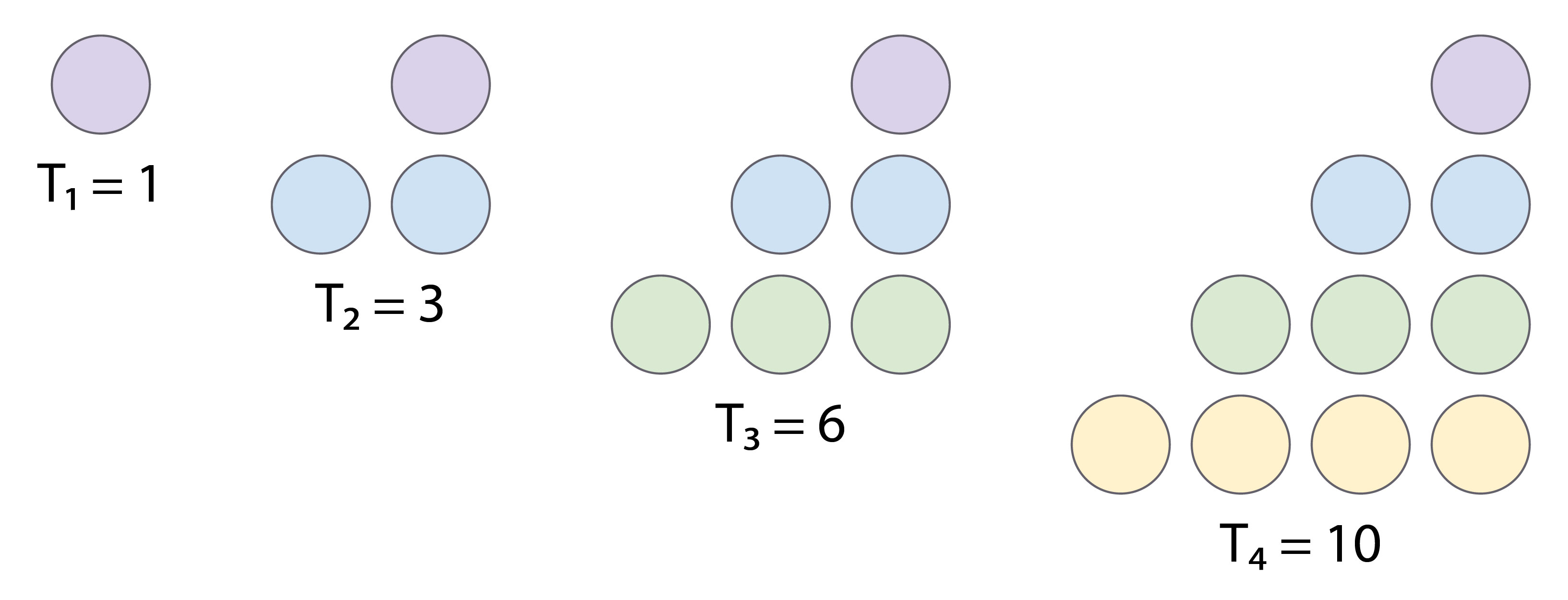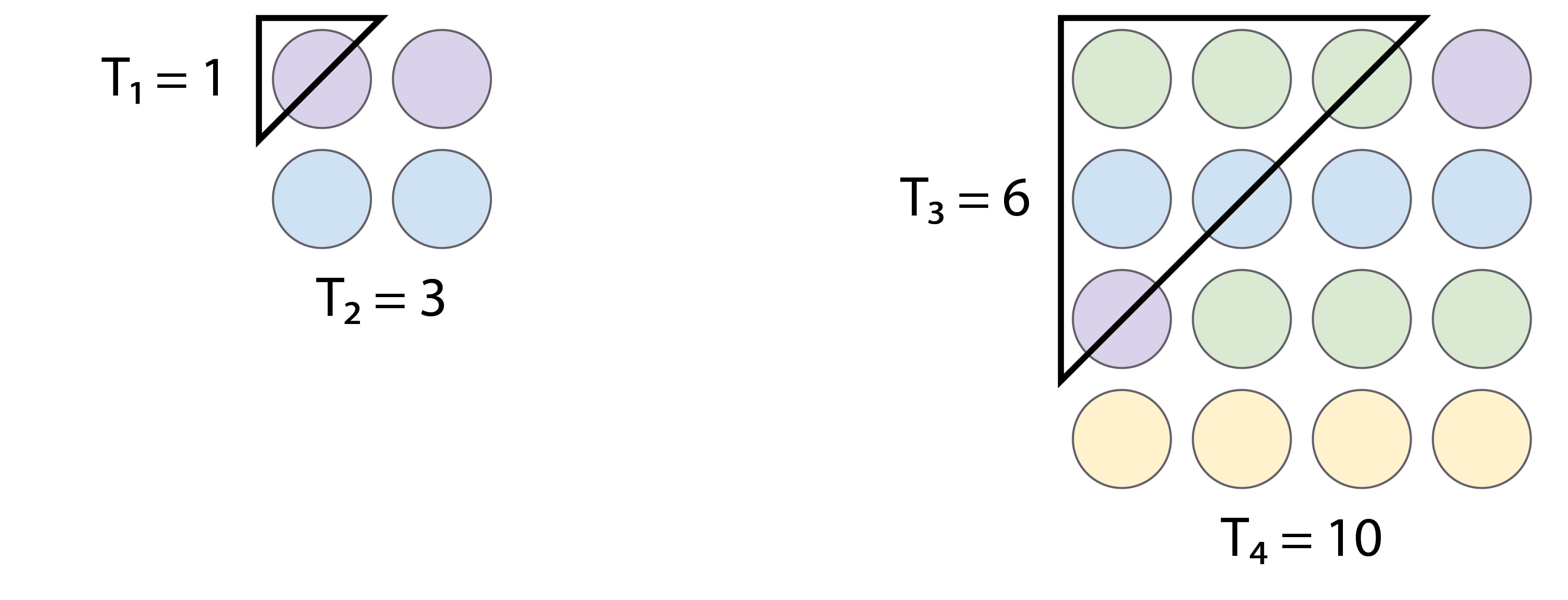# Blog

## Nifty triangle numbers

By

,

Difficulty: Fun

Triangular numbers are numbers that can be formed into a triangle.

For example, 3 is a triangular number with 2 dots on the bottom row and 1 dot on top row forming an equilateral triangle of 3 dots. 6 is the next triangular number with 3 dots on the bottom, 2 dots in the middle, and 1 dot on top forming a triangle of 6 total dots. If we add 4 dots underneath 6’s triangle, we make a new, bigger triangle of 10 dots, like so:If we start with one as our first triangle, each triangle after that adds one more dot to the row beneath. Our second triangle adds 2 dots to the row beneath one dot to make 3.

Given the pattern described above, what is the 10th (T10) triangular number?

Bonus question: 2 consecutive (right after each other) triangular numbers always add up to a square number. For example, T1 + T2 = 1 + 3 = 4 (the square of 2). Can you use geometry to show why this happens?

Scroll down or click for a hint, or the answer!## Brainteaser hint

First, use the pattern described above or illustrated in the picture to find out how many dots will be on the bottom row of the T10 triangle. Then, ask how many dots will be on the next row above, and the one above that and so on.

For the bonus question: Remember that a square number of dots can be arranged in a square grid.

Try visualising the triangular numbers as right triangles so that the dots in each triangle look like a set of stairs, like this:The tenth triangular number, T10, is 55.

The best way to look at these triangles is one layer at a time. Starting at the bottom, each layer has one fewer dot than the last. When you reach the top, it only has only 1 dot.

The size of the base is quite simple – the first triangular number has a base of 1, the second a base of 2 and so on. Extending this pattern, the 10th triangular number will have a base of 10 dots.

The most straightforward way to find T10 is to add up the rows starting with 10. Each subsequent row will have one less dot in it than the previous row. So, adding up the rows looks like this:

T10 = 10 + 9 + 8 +7 + 6 + 5 + 4 + 3 + 2 + 1 = 55

Bonus question: Two consecutive (right after each other) triangular numbers always add up to a square because these 2 triangles literally fit together to make a square!

Remember that a square number is the result of any whole number multiplied by itself. We call it a square number because squares have equal sides and so the area of a square is always length times equal length. A number multiplied by itself. Examples of square numbers include 1, 4, 9, 16, 25, 36, and so on.

To see how 2 consecutive triangular number become a square number, first imagine the triangular numbers as right triangles. This way the dots of each triangle are arranged like a set of stairs:Next, imagine rotating the smaller triangle 180 degrees and fitting it into the larger triangle:Using geometry, we can see that 2 consecutive triangular numbers make a square. In other words, they add up to a square number. On the left we see that 1 and 3 fit together to make a 2×2 square where the dots add up to the square number 4 (or 22). On the right we see that 6 and 10 make a 4×4 square where the dots add up the square number 16 (or 42).

If you’re not convinced by the geometry, here’s an equation-based explanation. We’ll use the triangles of 8 and 7 as an example, but this method will work for any 2 consecutive triangle numbers.

T8 = 8 + 7 + 6 + 5 + 4 + 3 + 2 + 1
T7 = 7 + 6 + 5 + 4 + 3 + 2 + 1

We can add (+ 0) to the T7 formula so that both equations have 8 terms, and then re-order the sum from smallest to largest:

T7 = 0 + 1 + 2 + 3 + 4 + 5 + 6 + 7

And then we can add T8 and T7, term by term:

T8 + T7 = (8 + 0) + (7 + 1) + (6 + 2) … + (1 + 7)
T8 + T7 = 8 + 8 + 8 + 8 + 8 + 8 + 8 + 8
T8 + T7 = 8 x 8

Categories:

## Similar posts

This site uses Akismet to reduce spam. Learn how your comment data is processed.

By posting a comment you are agreeing to the Double Helix commenting guidelines.